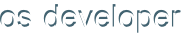View unanswered posts | View active topics It is currently Fri Nov 15, 2019 6:31 pm

 Print view Previous topic | Next topic
The difference between 16, 32, 64 bits.
Author Message

Joined: Mon Feb 08, 2010 12:20 pm
Posts: 12Hey there,

I don't really get the 16/32/64 bit memory. Why does 65535 is the maximum for 16 bits and also for 32 bits, I'm sure that's no true, however, I don't really understand this. Theoretically if in 16 bits maximum is FFFF, then in 32 it should be FFFF FFFF (131070). If I remember right then the old MS DOS (16 bit), couldn't recognize files larger than 4GB, but FAT 32 (for example Windows XP), can see and use this information, but cannot copy files that are larger than 4GB, where's the sense and what's the meaning of this?

Also, could some one of you, please, share with me some see-through ASM examples 16/32/64 bit versions and how would they look like in machine code. That would help me, it's easier to me to have a talk-through with people, rather than reading encyclopedias.

Thanks a lot.

Wed Feb 17, 2010 5:10 pmJoined: Tue Jan 19, 2010 11:51 pm
Posts: 662^16
2^32
2^64

Wed Feb 17, 2010 6:16 pmJoined: Tue Jul 28, 2009 4:09 am
Posts: 58
Location: United KingdomHey,

The Binary numeral system is a format for representing numbers in positional notation with a radix 2. That is, for each number from right to left in the number, the value it represents increases by a power of 2; in the same way that adding zeroes in normal (base-10) numbering increases in 10-fold. This is the common decimal system you know, which counts 1 to 9, then 10. In this system, "10" represents the value ten. In Binary, "10" represents the value 2, because the second position is worth one amount of 2's.

This system is representied electronically in binary computer busses by having 2 values for each line on a bus. So a 32-bit bus, has 32 lines, each representing a gradually increasin power of 2.

Now, as you know, 11 in decimal is the value eleven. However 1111 is not the value twenty-two. It is the value one thousand one hundred and eleven. The same goes for binary, and hexidecimal. Hexedecimal (the format you use when writing FFFF, is a positional notational, radix 16; so each position counts 1 to 9, and then A to F. The Value FFFF FFFF, is not simply two times FFFF, it is 65537 (10001) times larger.

Hexedecmial can easily be used to represent binary values because 16 is a power of 2. That means that there is a position in the binary notation (2^4, 2 to the power 4) that is equal to a position in hexedecimal (16^1). No such position appears in decimal, so it is difficult to convert between the two. This means that the any value stored in only 4 hexedecimal digits (FFFF) is equal to that which can be stored in 16 binary digits (1111111111111111).

So the maximum value that can be stored in 16-bits (16 binary digits) is 65535 (there are 65536 possible values including 0). That value is equal to 1 in al 16 positions or...
Code:
2^0+2^1+2^2+2^3+2^4+2^5+2^6+2^7+2^8+2^9+2^10+2^11+2^12+2^13+2^14+2^15

Which is the same as simply
Code:
2^16-1

A 32-bit number continues the pattern, so it's maximum value is 4294967295 (2^32-1).

Thanks,

James

Wed Feb 17, 2010 6:33 pm
Display posts from previous:  Sort by

#### Who is online

Users browsing this forum: No registered users and 1 guest

 You cannot post new topics in this forumYou cannot reply to topics in this forumYou cannot edit your posts in this forumYou cannot delete your posts in this forumYou cannot post attachments in this forum

Search for:
 Jump to:  Select a forum ------------------ Beginners    Hardware    Programming Languages    Computer Theory Advanced    Hardware    Programming Languages    Computer Theory BF Website    General    Bona Fide Network Projects General